In our previous article, we were discussing features, pin diagrams, internal features of the KS0108 controller based GLCDs and graphic LCD interfacing with a microcontroller. In this article, we are discussing the implementation of different instructions.

## Instructions in KS0108 Controllers

KS0108 controllers have seven instructions, they are given in the following table.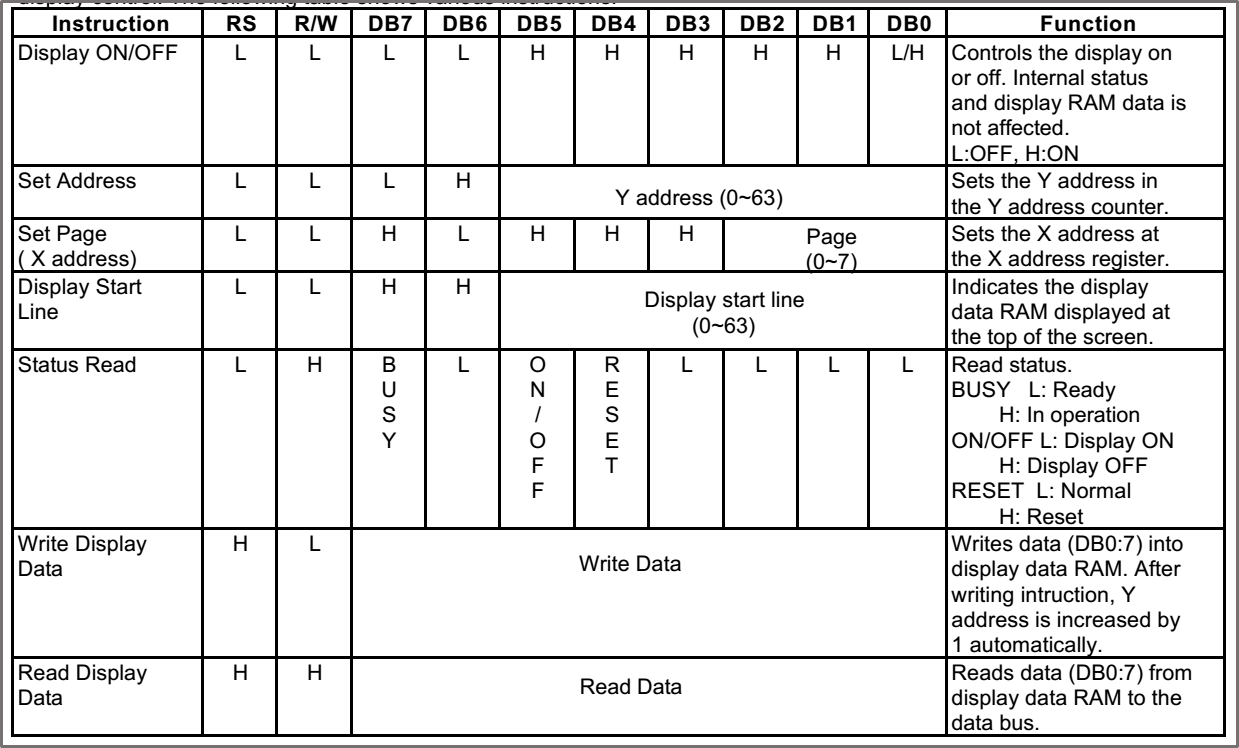The enable pin works as a clock for the GLCD. During write instructions, after setting each pin of the corresponding value, enable pin must be applied with a high to low pulse. The data will be latched at the falling edge of the E. For reading instructions, data appears while E is at a high level. Always give a delay of more than Ecycle (refer datasheet of the KS0108) between each instruction and also consider other timing parameters like delay time, rise time and setup time. Refer the timing diagram of write and read cycles in the datasheet.
Another important thing to consider is, most instructions are write instructions and some are in reading mode. So we will need to set the microcontroller port direction accordingly.

## Write Command

Below is the write timing diagram of the KS0108 controller. In the diagram, most important characteristics are illustrated. They are Tc (E cycle time), Twl(E Low-level width time), Twh(E High-level width time), Tasu (Address set-up time), Tdhw (Data hold time – write). There are other characteristics like rise time, fall time and address hold time. They are not included in the firmware because they are either covered by other characteristics or they are not that significant. Please refer the KS0108 datasheet for finding out the maximum, minimum and typical values of the timing parameters.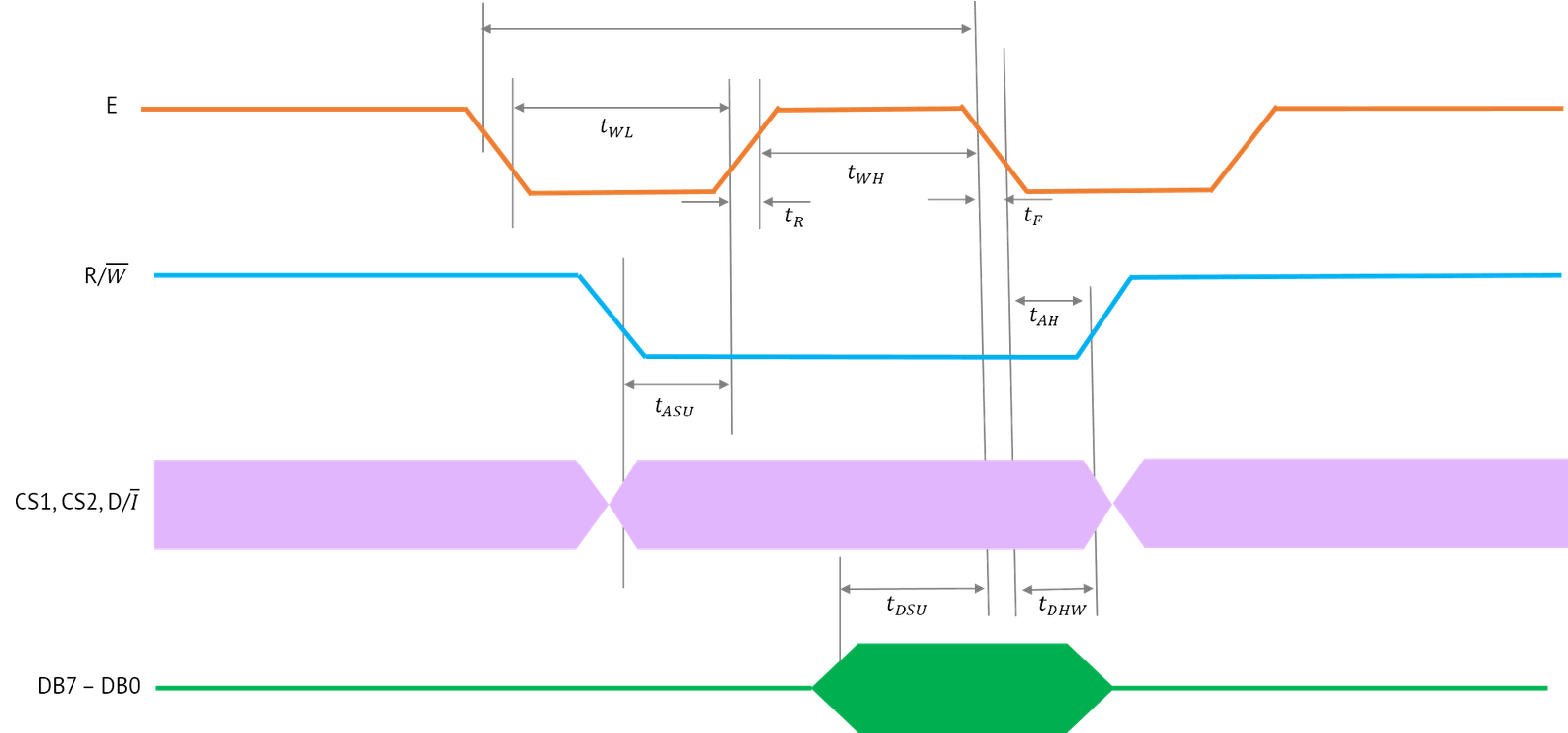Using the above timing diagram, we can create a common function to write command or data.

```// r: 0 for command and 1 for data
void GLCD_Write (unsigned char r, unsigned char data)
{
GLCD_Data_Direction = 0x00; // Set as Output
GLCD_E = 0;         // E pin Low
GLCD_DI = r;        // RS(DI) set low for command or high for data
GLCD_RW = 0;        // RW set low for write mode
delay_us(1);        // Twl > 450ns & Tasu > 140ns
GLCD_Data = data;   // Set dat in the data port
GLCD_E = 1;         // E pin High
delay_us(1);        // Twh > 450ns and Tdsu > 520ns
GLCD_E = 0;         // E pin Low
delay_us(1);        // Tdhw > 10ns
GLCD_E = 1;         // E pin High
}```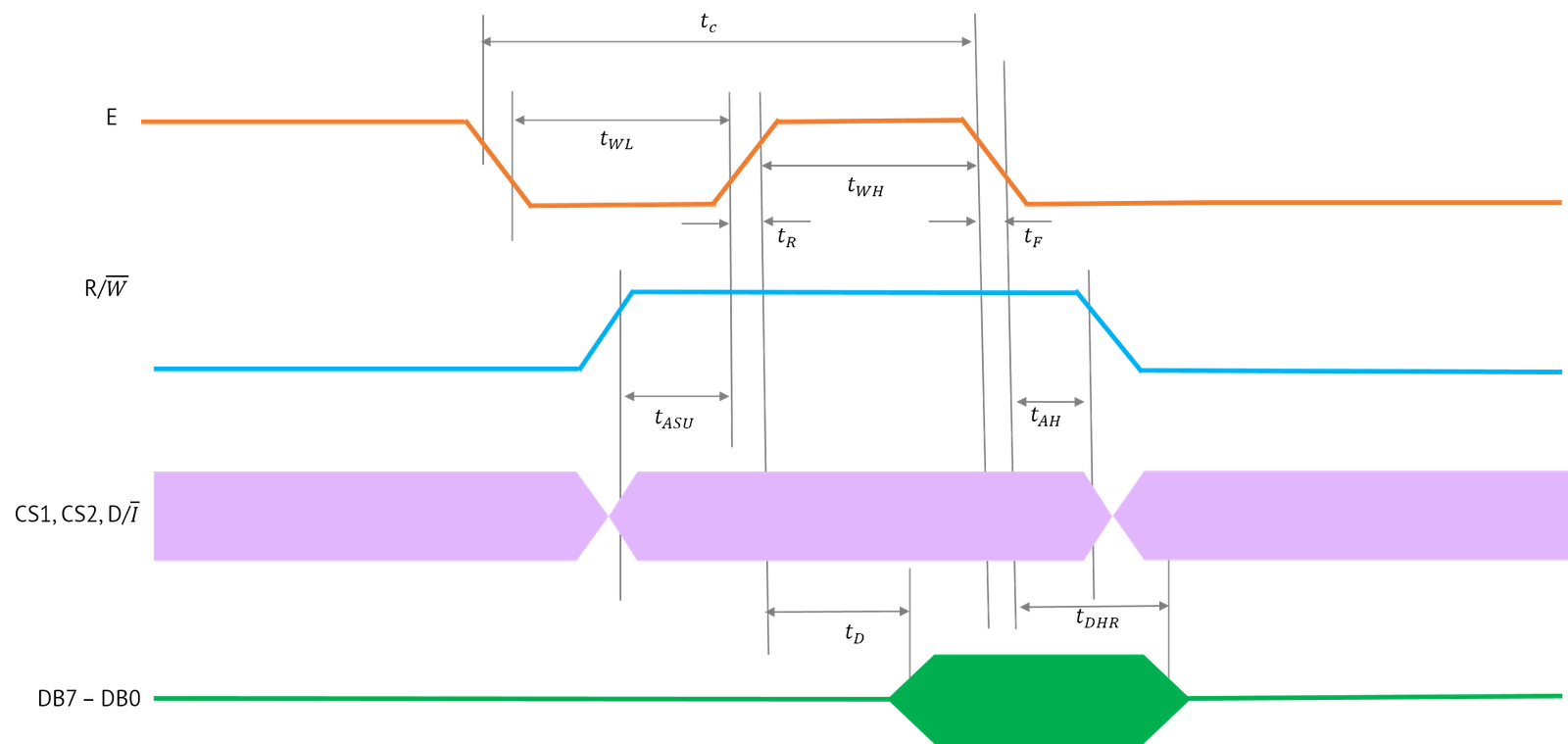From the above timing diagram, we can write the function for reading data as below.

```unsigned char GLCD_Read (unsigned char r)
{
unsigned char data;
GLCD_Data_Direction = 0xFF; // Set as Input
GLCD_E = 0;         // E pin Low
GLCD_DI = r;        // RS(DI) set low for command or high for data
GLCD_RW = 1;        // RW set low for read mode
delay_us(1);        // Twl > 450ns & Tasu > 140ns
GLCD_E = 1;         // E pin High
delay_us(1);        // Data appears after a delay of Td (>320ns)
// read data after considering E High level width time Twh > 450ns
// 500ns is enough
data = GLCD_Data;   // Set data in the data port
GLCD_E = 0;         // E pin Low
delay_us(1);        // Tdhr > 20ns, before disappearing data in the line
}```

## KS0108 Instructions

### Display On

This is the first and foremost command to set during the initialization. This instruction will turn ON the display. The above command is translated to the code as below.

```void GLCD_Init()
{
GLCD_Reset = 1;     // Reset the display
GLCD_CS1 = 1;       // Select left controller
GLCD_CS2 = 1;       // Select right controller
GLCD_Write(0, 0x3F); // Write command for turning ON
}```

This instruction sets the Y address of the display. If the address is less than 64, it is controlled by left. If it is between 64 and 128, it is on the right controller. So we need to turn ON the correct controller based on the display address.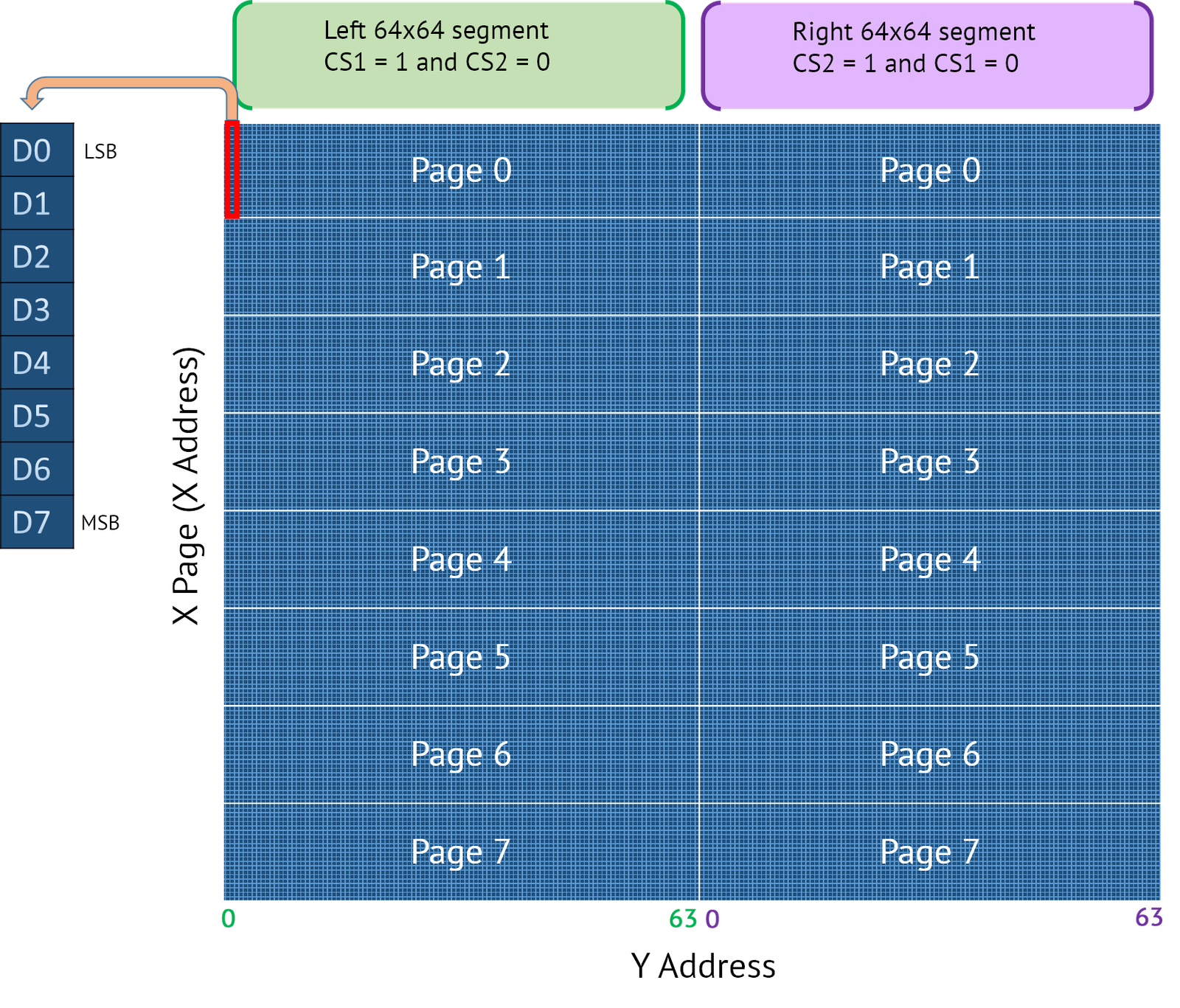```void Set_Column(unsigned char col)
{
unsigned char data;
if (col<64)
{
GLCD_CS1 = 1;   // Turn ON left controller
GLCD_CS2 = 0;
// Command format  -> 01XXXXXX
// XXXXXX -> Column address in 0 - 63 range
data = (col | 0x40) & 0x7F;
GLCD_Write(0,data);
}
else
{
GLCD_CS1 = 0;
GLCD_CS2 = 1;   // Turn ON right controller
// Command format  -> 01XXXXXX
// XXXXXX -> Column address in 0 - 63 range
data = ((col-64) | 0x40) & 0x7F ;
GLCD_Write(0,data);
}
}```

## Set Page (Set Row)

The display pointer can be set to a row with the help of simple instruction. Put the instruction on data port and give a high to low pulse on enable pin.

```void Set_Row(unsigned char row)
{
unsigned char data;
// Command format :10111XXX, XXX -> row
data= (row | 0xB8) & 0xBF;
GLCD_Write(0,data);
}```

By combining the above two functions, we can create a function to set the XY position.

```void Set_XY(unsigned char row, unsigned col)
{
Set_Row(row);
Set_Column(col);
}```

## Display Start Line

This instruction sets the starting line of the display from the display data RAM. For example, if you want to roll the content, set the start line as first, then set it as second and so on. The viewer will see the content on the display RAM’s first page on the first line, then data from the second page on the first line (after setting it as second using the display start line instruction) and so on. This instruction can be used for animating the content.

```void Display_Set_Start_Line(unsigned char line)
{
unsigned char data;
GLCD_CS1 = 1;       // Select left controller
GLCD_CS2 = 1;       // Select right controller
data = 0xC0 | line;   // Set start line command
GLCD_Write(0,data);
}```

To check whether the display is busy/ON/reset, we can use the status read instruction. The busy flag indicates the display controller is operating or non-operating mode. If it is high, we need to wait till the flag becomes low.

```unsigned char GLCD_Busy()
{
unsigned char data;
return (data &&0x80);   // Return flag status,
//  1 if Busy, else 0
}

// function to wait until busy signal clear off
// This can be called after an instruction to wait till it clears busy flag
void Check_GLCD_Busy()
{
while GLCD_Busy();
}```

Below function checks whether the display is in reset condition or OFF. It returns 1 if it is OFF, 2 if it is reset.

```char GLCD_Status ()
{
unsigned char data;
return (data && 0x30);   // Return flag status,
//  1 if display OFF, 2 if reset and 0 else
}```

## Write Display Data

Write display data instruction writes a single byte of data from the data bus into the current address of the display RAM.

```void GLCD_Write_Char(unsigned char data)
{
GLCD_Write(1, data);    // Write in data mode
}

//Function to send multiple bytes to the RAM
// Make sure that data sent doesn't exceeds the page and Column limits
void GLCD_Write_Str(unsigned char *str, unsigned char count)
{
unsigned char c=0;
do{
GLCD_Write(1, str);    // Write in data mode
c++;
str++;
} while(c < count);
}```

This instruction reads the data from the current address of display RAM.

```char GLCD_Read()
{
unsigned char data;
return data;
}```

## Other Functions

Other functions that can be derived from the above functions are given below.

### Clear line

This function clear a given line.

### Clear display

This is for clearing the entire display contents.

```void GLCD_Clear_Display()
{
unsigned char i;
for(i=0;i<8;i++)
GLCD_Clear_Line(i);    // Clear each line
}```

## Fill Data

This function can be used to fill with same data all over the display.

```void GLCD_Fill(unsigned char data)
{
unsigned char i, j;
for(i=0,j=0;(i<8)&&(j<128)
{
Set_XY(i, j);
GLCD_Write_Char(data);
}
}```

## Display Dot

Below function displays a dot after setting position in x,y format.

```void GLCD_Dot(unsigned char x, unsigned char y)
{
Set_XY(x, y);    // Set position
GLCD_Write(1, 0x70);    // Write in data mode
}```

## Example Code Using Above Functions

Using the above-defined functions, we can write a sample code for a 128×64 display as below. Include above functions in the main file.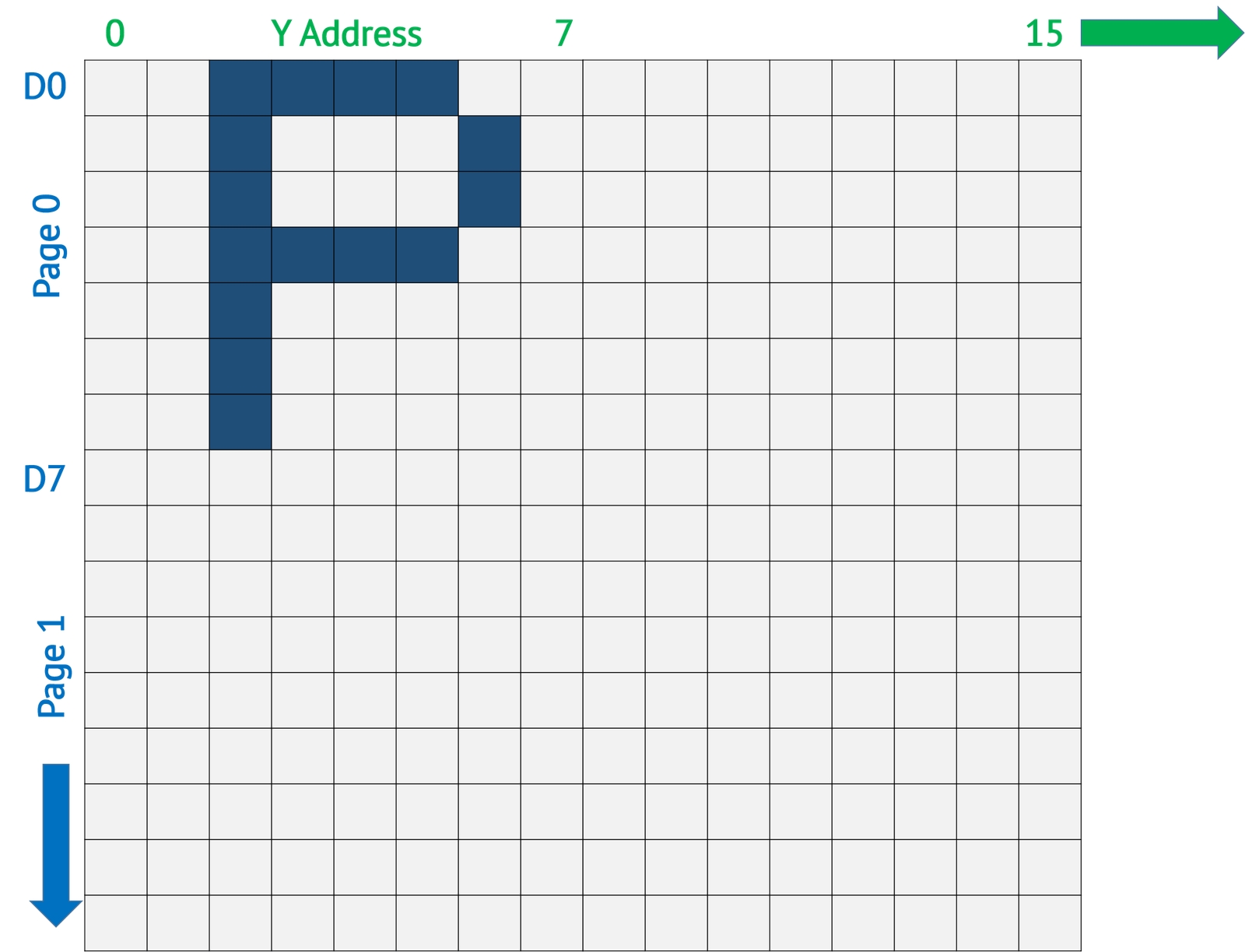Here we are going to display the letter “P” in (0,0) position of the display.

```#include <xc.h>
#include "glcd.h"

#define GLCD_Data = PORTD;
#define GLCD_Data_Direction = TRISD;
#define GLCD_E RA0;
#define GLCD_RW RA1;
#define GLCD_DI RA2;
#define GLCD_CS1 RA3;
#define GLCD_CS2 RA4;
#define GLCD_RST RA5;

void main()
{
// The character "P" in 5x7 font.
char str[] = {0x00, 0x00, 0x7F, 0x09, 0x09,0x09,0x06,0x00};
ADCON1 = 0x0F;      // Disable analog pins
TRISA = 0x00;       // 6 control lines as outputs
GLCD_Init();        // Turn ON
Check_GLCD_Busy();  // Wait if busy
GLCD_Clear_Display();
Set_XY(0,0);        // set pointer at 0,0
GLCD_Write_Str(str, 8); // Write "P" into the data memory
while(1);               //wait here
}```

We can create graphics like lines, circles and boxes and different sized fonts in a similar manner. In the next part of the tutorial, we are going to use a generic library which has all the features including graphics, fonts etc.